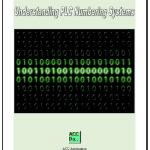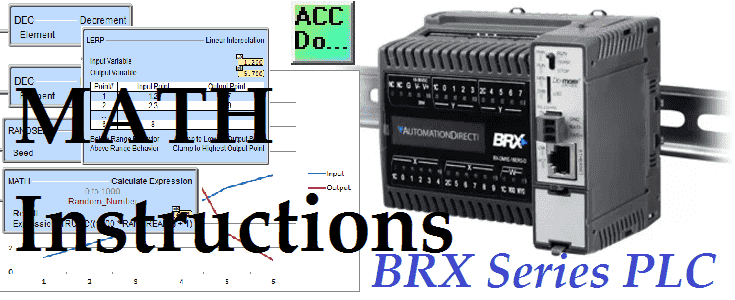# BRX Do-More PLC Math Instructions

Math instructions are used to perform mathematical calculations. The BRX Do-More PLC has math instructions that can be used in a wide variety of applications. We will be looking at the INC increment, DEC decrement, LERP linear Interpolation, RANDSEED Random Number Seed, and the MATH Calculated Expression instructions. The automation system that you implement may involve some or all of these instructions. As a system integrator, you will require the use of these instructions in your commissioned programs.
Let’s get started with the BRX Do-More PLC Math Instructions.

Previously in this BRX Do-More series PLC, we have discussed:
System HardwareVideo
Unboxing – Video
Installing the SoftwareVideo
Establishing CommunicationVideo
Firmware Update – Video
First ProgramVideo
Monitoring and Testing the ProgramVideo
Online Editing and Debug ModeVideo
TimersVideo
CountersVideo
High-Speed IOVideo
Compare InstructionsVideo

The best way to look at the math instructions is through examples. We will look at each of the instructions individually.

##### INC – Increment – BRX Do-More Math Instructions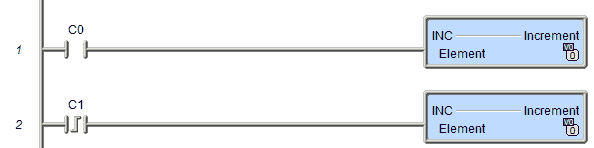The increment will add 1 to register V0 when the contact C0 is on. This increment by 1 will happen every scan as long as input bit C0 before it is on. If we need to activate this instruction only once then use a leading or trailing edge one-shot contact before the instruction. You can see that we have used a leading-edge one shot from C1 to increment V0 only once from a transition from off to on.

##### DEC – Decrement – BRX Do-More Math Instructions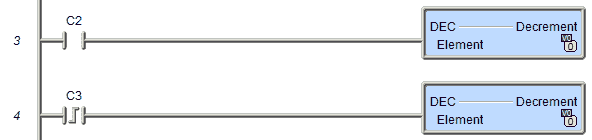The decrement will subtract 1 from register V0 when the contact C2 is on. This decrement by 1 will happen every scan as long as input bit C2 before it is on. If we need to activate this instruction only once then use a leading or trailing edge one-shot contact before the instruction. You can see that we have used a leading-edge one shot from C3 to decrement V0 only once from a transition from off to on.
Note: V0 is an unsigned word memory location. The unsigned word that can hold a value of 0 to 65535.

##### LERP – Linear Interpolation – BRX Do-More Math Instructions

The Linear Interpolation (LERP) instruction uses a table of up to 50 Input / Output data pairs. It then uses the data points in that table to interpolate or extrapolate an output value for any given input value.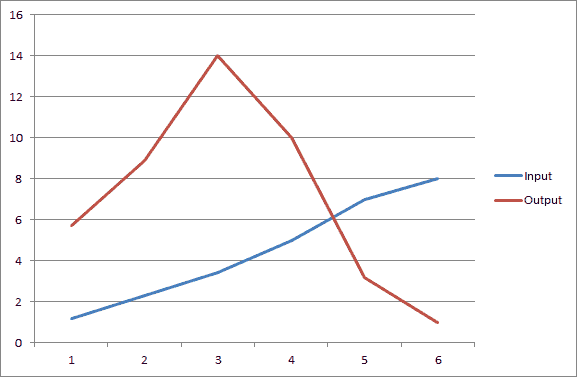In the above graph, we have six pairs of data. This represents a nonlinear expression. We can now enter this information in the linear interpolation instruction.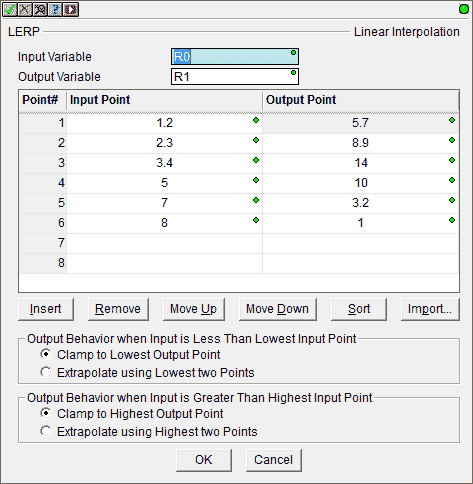Our input variable is R0. This is a real number. Our output variable that will be set is R1. The input points are written in the instruction itself. We also have the ability to set the output behavior when the input is less than or greater than the input points. In both of these cases, we have set this to clamp to the lowest and highest point.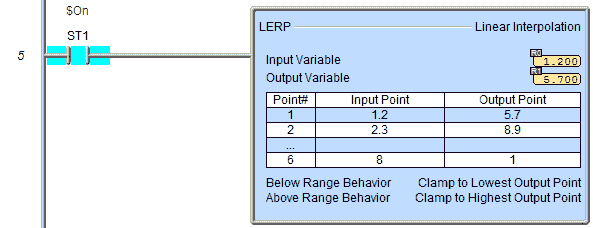We have used the always-on system bit ST1 as an input to this instruction. When we change the input in R0 to 1.5 the instruction will produce an output in R1 of 6.573. It will look at the table and extract the value.

##### RANDSEED – Random Number Seed – BRX Do-More Math Instructions

The Random Number Seed (RANDSEED) instruction sets a seed value that will be used by the Generate Random Integer (RANDINT) and Generate Random Real (RANDREAL) math functions.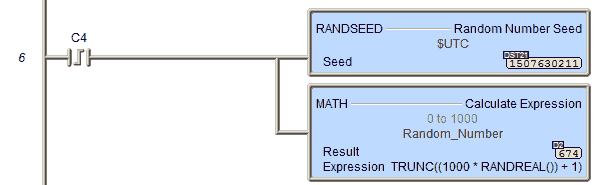A random seed is a number used to initialize a number generator. These two pseudo-random number generating instructions will always produce the same sequence of random numbers when initialized with that seed. A good choice for a random seed is the current UTC Coordinated Universal Time value (\$UTC (DST21)).
In our example, we are looking for a random number between 0 and 1000. The math expression to do this is the following:
TRUNC((1000 * RANDREAL()) + 1)
This is similar to an excel expression. =TRUNC((1000 * RAND() + 1)

##### MATH – Calculated Expression – BRX Do-More Math Instructions

The result of the instruction is designated a location to store the results of the math computation. This can be any writable numeric location or array reference. As we saw above this is similar to the formulas in excel.
The expression contains the memory locations, mathematical operators, and /or math functions from the following tables that make up the formula to calculate. This can be any valid combination of constant values, bit or numeric memory locations, math operators, nested parentheses, and math functions up to a maximum of 1024 characters. Expressions can be nested up to 8 levels deep and chained between operators within function parameters, and as array indices, so the use of parentheses is recommended to ensure proper evaluation order.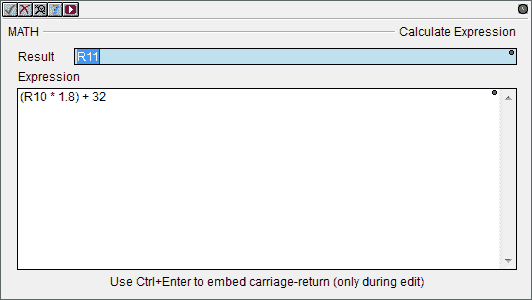In our example, we will be converting Celsius to Fahrenheit. We will be using the following formula.
Degrees F = (Degrees C * 1.8) + 32
The input Celsius C will come from R10. Our output (Fahrenheit Degrees) from the formula will be R11.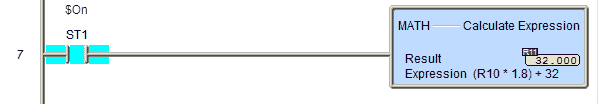If we input the value 0 degrees C into R10, our output will be 32 degrees F in R11.
The following tables will indicate all of the instructions that we can do in our math expression instruction.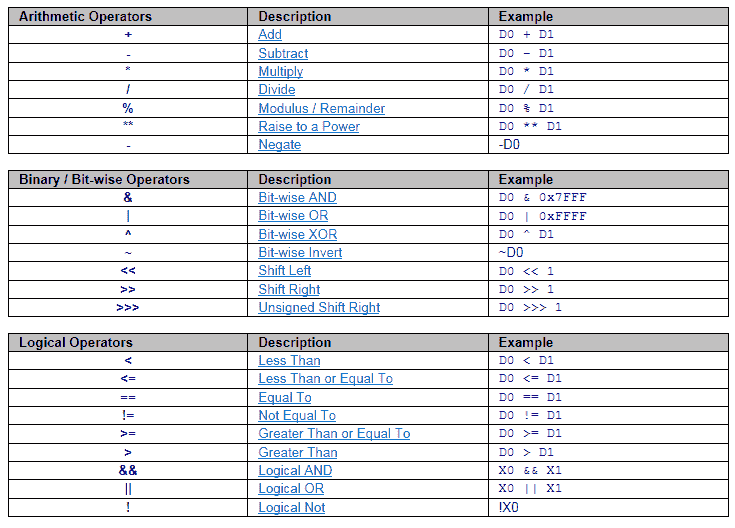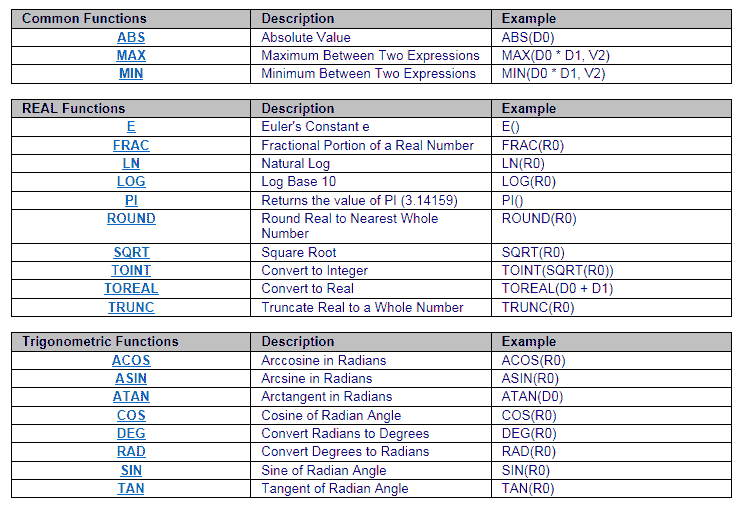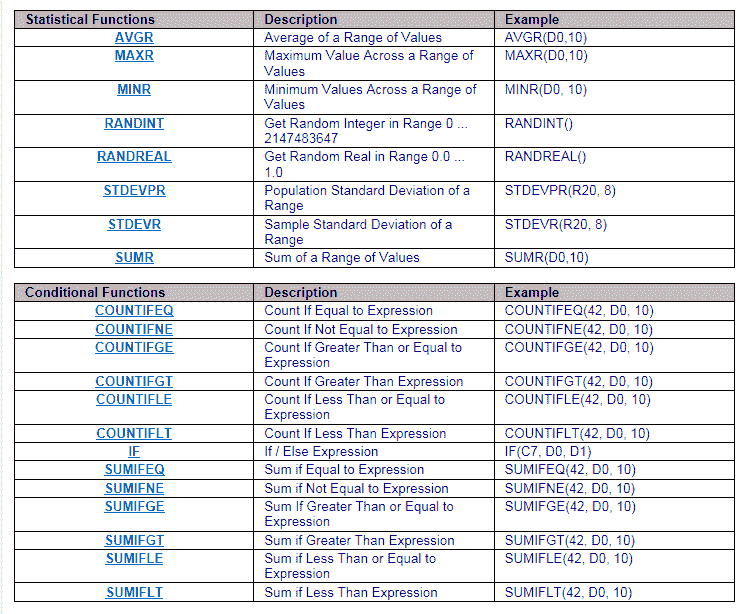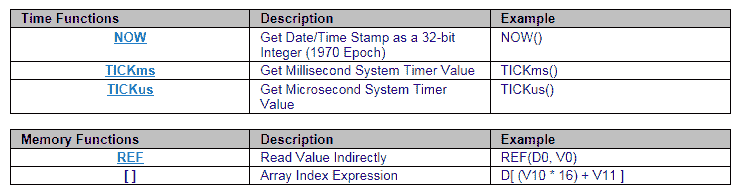You can see that the math capabilities of the BRX Do-More PLC is very extensive. Formulas are written in a straight forward way so we can concentrate more on our PLC logic. This ability is very important when determining your next controller for your automation project.

You can watch the video below to see how the math instructions work in the BRX Do-More Series PLC.

##### BRX Series PLC from Automation Direct – Power to deliver

Overview Link (Configure and purchase a system)
Manuals and Product Inserts (Installation and Setup Instruction)
Do-More Designer Software v2.0.3 (Free Download Link) – The software will contain all of the instruction sets and help files for the BRX Series PLC.

Next time we will look at program control in the BRX PLC.

Watch on YouTube : BRX PLC Math Instructions
Thank you,
Garry

If you’re like most of my readers, you’re committed to learning about technology. Numbering systems used in PLC’s are not difficult to learn and understand. We will walk through the numbering systems used in PLCs. This includes Bits, Decimal, Hexadecimal, ASCII and Floating Point.

To get this free article, subscribe to my free email newsletter.

Use the information to inform other people how numbering systems work. Sign up now.